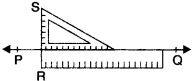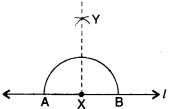NCERT Solutions for Class 6 Maths Chapter 14 Practical Geometry Ex 14.4 are part of NCERT Solutions for Class 6 Maths. Here we have given NCERT Solutions for Class 6 Maths Chapter 14 Practical Geometry Ex 14.4.

 Board CBSE Textbook NCERT Class Class 6 Subject Maths Chapter Chapter 14 Chapter Name Practical Geometry Exercise Ex 14.4 Number of Questions Solved 3 Category NCERT Solutions

## NCERT Solutions for Class 6 Maths Chapter 14 Practical Geometry Ex 14.4

Question 1.
Draw any line segment $$\overline { AB }$$ . Mark any point M on it. Through M draw a perpendicular to $$\overline { AB }$$. (use ruler and compasses).
Solution :
Step 1. Given a point M on any line $$\overline { AB }$$.Step 2. With M as centre and a convenient radius, construct a part circle (arc) intersecting the line segment $$\overline { AB }$$ at two points C and D.
Step 3. With C and D as centres and a radius greater than CM, construct two arcs which cut each other at N.
Step 4. Join $$\overline { MN }$$. Then $$\overline { MN }$$ is perpendicular to $$\overline { AB }$$ at M, i.e., $$\overline { MN }$$ $$\overline { AB }$$.

Question 2.
Draw any’line segment $$\overline { PQ }$$. Take any point R not on it. Through R draw a perpendicular to $$\overline { PQ }$$. (use ruler and set-square).
Solution :
Step 1. Let $$\overline { PQ }$$ be the given line segment and R be a point not on it.Step 2. Place a set-square on $$\overline { PQ }$$ such that one arm of the right angle aligns along $$\overline { PQ }$$.
Step 3. Place a ruler along the edge opposite of the right angle.
Step 4. Hold the ruler fixed. Slide the set-square along the ruler all the point R touches the arm of the set-square.
Step 5. Join RS along the edge through R, meeting $$\overline { PQ }$$ at S. Now $$\overline { RS }$$ $$\overline { PQ }$$.

Question 3.
Draw a line l and a point X on it. Through X, draw a line segment $$\overline { XY }$$ perpendicular to l. Now draw a perpendicular to XY at Y. (use ruler and compasses)
Solution :
Step 1. Given a point X on a line l.
Step 2. With X as centre and a convenient radius, construct a part circle (arc) intersecting the line l at two points A and B.
Step 3. With A and B as centres and a radius greater than AX, construct two arcs which cut each other at Y.Step 4. Join $$\overline { XY }$$. Then $$\overline { XY }$$ is perpendicular to l atX, i.e., $$\overline { XY }$$ l.

We hope the NCERT Solutions for Class 6 Maths Chapter 14 Practical Geometry Ex 14.4 help you. If you have any query regarding NCERT Solutions for Class 6 Maths Chapter 14 Practical Geometry Ex 14.4, drop a comment below and we will get back to you at the earliest.